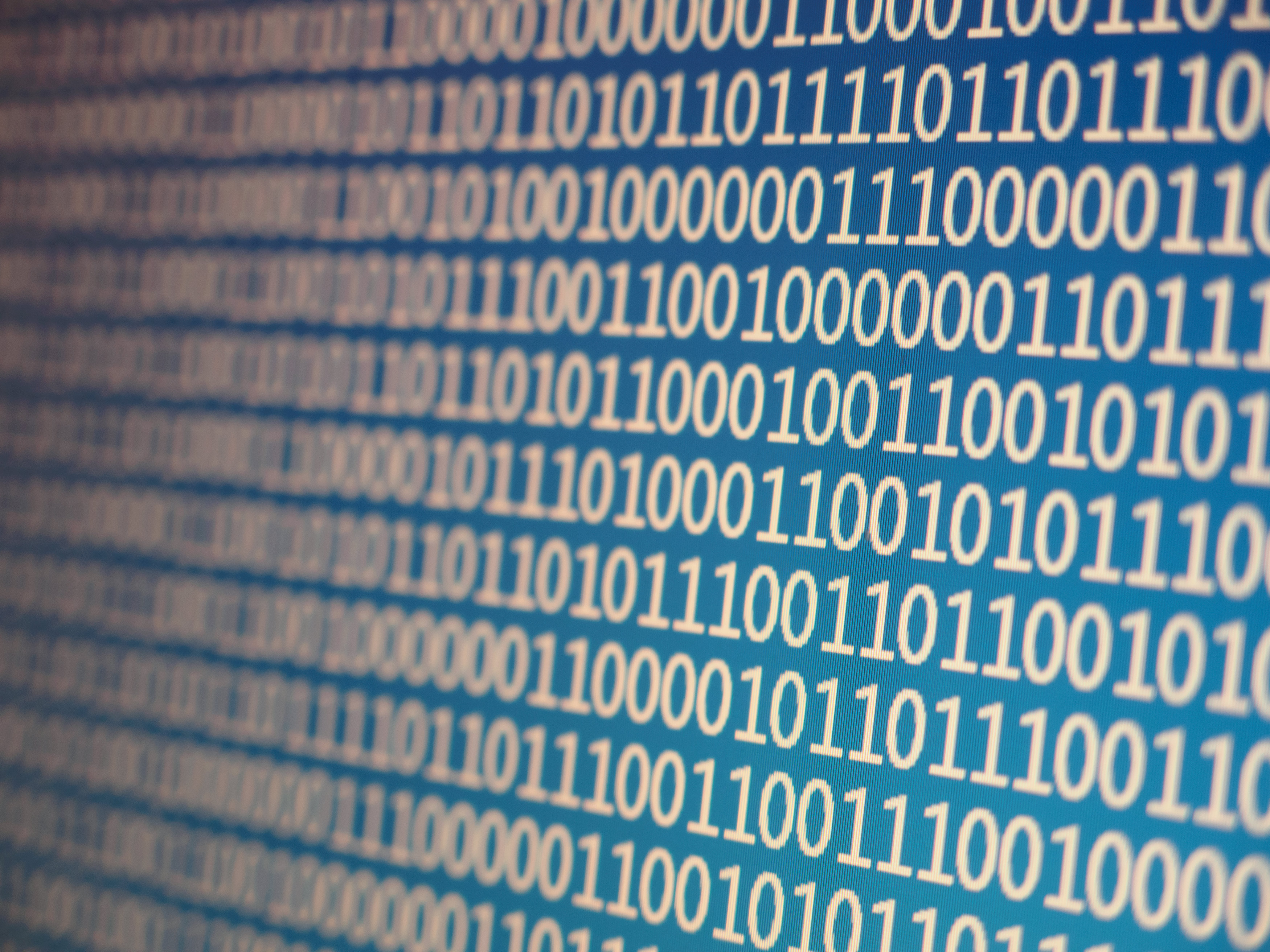# Binary Facts for Kids## So, What Exactly Is Binary?

Binary, also known as the base-2 number system, is a way of writing numbers by using only two different symbols, usually a one (1) and a zero (0). Even though this is a whole eight symbols less than we normally use (this is called decimal, or the base-10 number system), it's still possible to write out each and every number.

While counting with only two symbols can seem pretty confusing for humans, binary makes a lot of sense when thinking about computers. In fact, binary is used in just about every computer on Earth and every time you've used one, you've seen binary in action.

## Numbers in Decimal

To get an idea of how binary works, let's first take a closer look at the decimal system (remember, the decimal system, or base-10, is how we normally write numbers). What happens when we count from nine to ten?

English Base-10
Nine 9
Ten 10
If you were paying close attention, you might've noticed that we only used one symbol (9) for the number nine, but had to use two (1 and 0) to write the number ten. We can keep track of each "place" (one's place, ten's place, etc.) only as long as we have enough symbols.

## Numbers in Binary

Okay, so here's where things start getting cool. Binary works in exactly the same way! The only difference is, we only have one symbol before we "run out" and have to start using another digit. Let's try counting again, this time from zero to two, and in both decimal and binary.

English Base-10 Base-2
Zero 0 0
One 1 1
Two 2 10
If you noticed, binary is the same as decimal until we reach the number two. Because we can't use the symbol 2 (or 3-9) anymore, we have to write two as 10. If we kept going, three would be written 11 and four would be 100. Starting to get it?

• ## The usefulness of binary code was understood centuries before the invention of the first digital computer.

In 1689, Gottfried Leibniz wrote an article explaining the basics of the binary numeral system and some of its potential uses. Although the idea for a programmable computing device had been put forward by Charles Babbage in the early 18th century, it wasn't until 1941 with Konrad Zuse's invention of the Z3, the first programmable, digital computer, that binary was put to use in computers.

• ## Binary numbers are stored in computers as groups of bits.

Bits are the smallest unit of storage in a computer. The word comes from combining the two words binary and digit. In a computer, a bit can either be on or off, representing either the binary digits 1 or 0.

To make longer binary numbers, several bits are needed. For example, the binary number 101 (5 in decimal) requires 3 bits. A byte is made up of 8 bits. Sound familiar?